# Polygon

The Polygon command draws a closed polyline with a specified number of sides.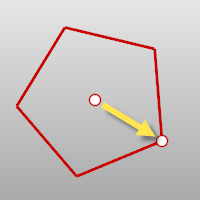Steps

Follow the prompts for the selected option. If no option is specified, the default option is used.

Polygon options

NumSides

The NumSides option specifies the number of sides for the polygon.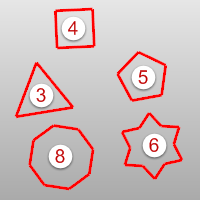Vertical

The Vertical option draws a polygon perpendicular to the construction plane.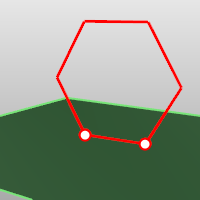AroundCurve

The AroundCurve option draws a polygon perpendicular to a curve.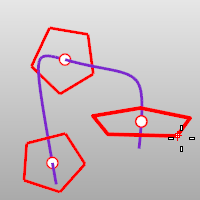Select a curve and pick the location on the curve and radius.

Inscribed

The Inscribed option draws the polygon where the corners of the sides touch an imaginary circle of a specified radius.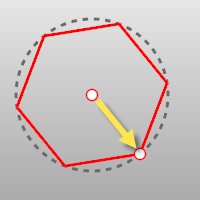Inscribed steps

 1 Pick the center.
 2 Pick a corner.

Circumscribed

The Circumscribed option draws the polygon where the midpoints of the sides touch an imaginary circle of a specified radius.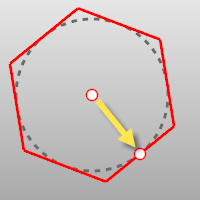Circumscribed steps

 1 Pick the center.
 2 Pick the midpoint of the polygon edge.

Edge

The Edge option draws a polygon by defining one edge.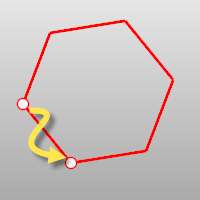Edge steps

 1 Pick the start of the edge.
 2 Pick the end of the edge.

Edge option

Flip

Flips the polygon about the edge you are drawing.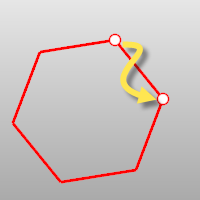Star

The Star option draws a star-shaped polygon.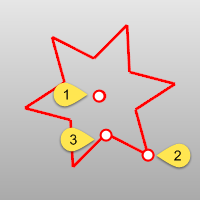Star steps

 1 Pick the center (1).
 2 Pick the corner (2).
 3 Pick the radius for the imaginary circle defining the second star radius (3).

Star option

Automatic

If the polygon has 5 or more sides, the Automatic option creates a star where the size of the outer and inner points are equal.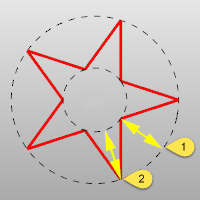Distances 1 and 2 are equal.